# Optimal System

## Optimal Scheduling of Hydrothermal Processes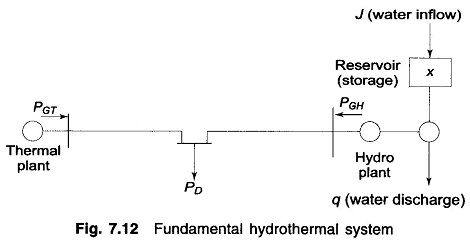Optimal Scheduling of Hydrothermal Processes: The earlier sections have dealt with the problem of optimal scheduling of a power system with thermal plants only. Optimal operating policy in this case can be completely determined at any instant without reference to operation at other times. This, indeed, is the static optimization problem. Operation of a system …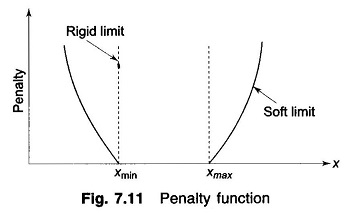Optimal Load Flow Solution: The problem of optimal real power dispatch has been treated in the earlier section using the approximate loss formula. This section presents the more general problem of real and reactive power flow so as to minimize the instantaneous operating costs. It is a static optimization problem with a scalar objective function …

## Derivation of Transmission Loss Formula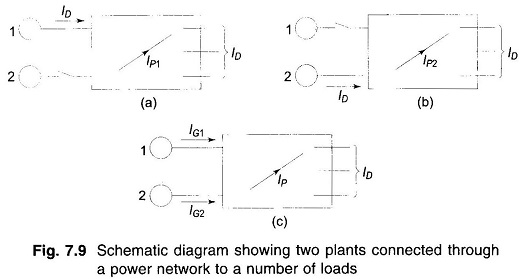Derivation of Transmission Loss Formula: An accurate method of obtaining a general derivation of transmission loss formula has been given by Kron. This, however, is quite complicated. The aim of this article is to give a simpler derivation by making certain assumptions. Figure 7.9 (c) depicts the case of two generating plants connected to an …

## Optimum Generation Scheduling

Optimum Generation Scheduling: Optimum Generation Scheduling – From the unit commitment table of a given plant, the fuel cost curve of the plant can be determined in the form of a polynomial of suitable degree by the method of least squares fit. If the transmission losses are neglected, the total system load can be optimally …

## Reliability Consideration in Power System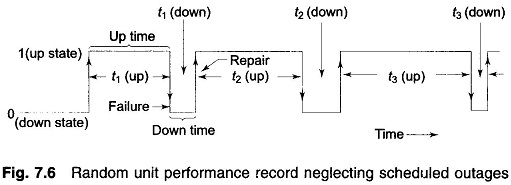Reliability Consideration in Power System: With the increasing dependence of industry, agriculture and day-to-day household comfort upon the continuity of electric supply, the Reliability Consideration in Power System has assumed great importance. Every electric utility is normally under obligation to provide to its consumers a certain degree of continuity and quality of service (e.g. voltage …

## Optimal Operation of Generators on a Bus Bar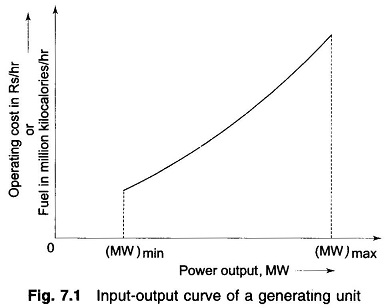Optimal Operation of Generators on a Bus Bar: Optimal Operation of Generators on a Bus Bar – The major component of generator operating cost is the fuel input/hour, while maintenance contributes only to a small extent. The fuel cost is meaningful in case of thermal and nuclear stations, but for hydro stations where the energy …

## Introduction to Optimal System Operation

Introduction to Optimal System Operation: The optimal system operation, in general, involved the consideration of economy of operation, system security, emissions at certain fossil-fuel plants, optimal releases of water at hydro generation, etc. All these considerations may make for conflicting requirements and usually a compromise has to be made for optimal system operation. Here we …

Scroll to Top